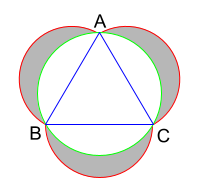# Practice on Toph

Participate in exhilarating programming contests, solve unique algorithm and data structure challenges and be a part of an awesome community.

# Bored in Box

By AlbinHossain · Limits 1s, 512 MB

After becoming a demon, Nejuko can’t go out in the sunlight. Most of the daytime she stays in a box. Though she never complained, this is extremely boring to her. After all, why a demon (or human) would like to live in such a tiny box alone! That's why her elder brother Tanjiro decides to give her a problem to solve to bring some excitement.

Tanjiro drew an equilateral triangle $ABC$ with its circumcircle. Then he drew three half circles each based on exactly the sides of the triangle. Finally, he got a figure like below.Now Tanjiro gives the length of the side $AB$ of the triangle and asks Nejuko to find out the area of the dark shaded region.

After trying for some times, Nejuko was almost close to the solution, she derived that the area of the given triangle is equal to $\frac{\sqrt{3}}{4}BC^2$ and circumradius of the given triangle is equal to $\frac{BC}{\sqrt{3}}$.

After doing this much stuff Nejuko started feeling tired. She needs to sleep now to gain some energy. Before going to sleep she asked you to solve the same problem.

She also said one more thing, “You must use $3.14159$ for the value of $pi$$(\pi)$”.

## Input

The input will contain only one integer $AB$, the length of the one side of the triangle.

$1\leq AB \leq 3\times10^4$

## Output

You have to output the area of dark shaded region. Your answer is considered correct if its absolute or relative error doesn't exceed $10^{-6}$.

## Samples

InputOutput
14

110.526807904208283

InputOutput
6

20.300842268119887


Notes

• An equilateral triangle is a triangle in which all three sides of the triangle have the same length.

• Circumcircle of a triangle is the minimum radius circle that passes through all the vertices of that triangle. Circumradius is the radius of Circumcircle.

• Half circles are exactly based on the sides of the triangle means the diameters of those half circles are equal to the respective sides of the triangle.

### Statistics

100% Solution Ratio

MursaleenEarliest, 1w ago

MursaleenFastest, 0.0s

MursaleenLightest, 0 B

bokaifShortest, 75B

### Submit

Login to submit

### Editorial

If we subtract the area of the circumcircle from the summation of area of triangle and area of the h...

### Related Contests

 DIU Take-Off Programming Contest Spring 2020Ended 1w ago